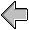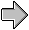# Complete Program from Figure 11

```(define Basic-Shapes
(unit (import)
(export Shape Rectangle Circle Translated)

(define Shape (interface () draw))

(define Rectangle
(class* object% (Shape) (width height)
(public
[draw (lambda (dc x y)
(send dc draw-rectangle x y width height))])
(sequence (super-init))))

(define Circle
(public
[draw (lambda (dc x y)
(send dc draw-ellipse
(sequence (super-init))))

(define Translated
(class* object% (Shape) (orig-shape dx dy)
(public
[draw (lambda (dc x y)
(send orig-shape draw
dc (+ x dx) (+ y dy)))])
(sequence (super-init))))))

(define Union-Shape
(unit (import Shape)
(export Union)

(define Union
(class* object% (Shape) (left right)
(public
[draw (lambda (dc x y)
(send left draw dc x y)
(send right draw dc x y))])
(sequence (super-init))))))

(define Basic+Union-Shapes
(compound-unit
(import)
[US (Union-Shape (S Shape))])
(export (S Shape)
(S Rectangle)
(S Circle)
(S Translated)
(US Union))))

(define Gui
(unit (import Shape canvas% frame%)
(export display-shape)

(define shape-canvas%
(class canvas% (parent shape)
(inherit get-dc)
(override
[on-paint
(lambda ()
(send shape draw (get-dc) 0 0))])
(sequence (super-init parent))))

(define display-shape
(lambda (a-shape)
(unless (is-a? a-shape Shape)
(error 'display-shape "expected a Shape, got: ~e" a-shape))
(let* ([frame (make-object frame% "Shapes" #f 150 150)]
[canvas (make-object shape-canvas% frame a-shape)])
(send frame show #t))))))

(define Picture
(unit (import Rectangle Circle Translated display-shape)
(export shape1 shape2)

(define shape1 (make-object Rectangle 40 30))
(define shape2 (make-object Translated
(make-object Circle 20)
30 30))

(display-shape shape1)
(display-shape shape2)))

;; the graphics library (mred@) is defaultly a signed unit.
;; see mzscheme manual for details of signed units.
(define MrEd-Toolkit (unit/sig->unit mred@))

(define Union-Picture
(unit (import Rectangle Circle Translated Union
shape1 shape2
display-shape)
(export)

(define shape3 (make-object Union shape1 shape2))
(display-shape shape3)))

(define Union-Program
(compound-unit
(import)
[M (MrEd-Toolkit)]
[G (Gui (S Shape) (M canvas%) (M frame%))]
[P (Picture (S Rectangle)(S Circle)(S Translated)
(G display-shape))]
[UP (Union-Picture (S Rectangle)
(S Circle)
(S Translated)
(S Union)
(P shape1)
(P shape2)
(G display-shape))])
(export)))

(invoke-unit Union-Program)

```figurein context contents